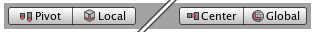# Object's vectors are not moving with the object, what did i do wrong?

i found a youtube video (Sebastian Lague) that has a really good movement script, i understood it and used it for my character, when i started moving it, i noticed that the character rotates but his blue, red, green vectors are not rotating with him, his forward always stays the same and it was annoying when i implemented rotation by mouse, also in the youtube video his vectors rotated fine with the object, what did i do wrong? please i need this.

`````` Vector2 input = new Vector2(Input.GetAxisRaw("Horizontal"), Input.GetAxisRaw("Vertical"));
Vector2 inputDir = input.normalized;

//if (inputDir==new Vector2 (0,1))
//    transform.eulerAngles = new Vector3(0, Camera.main.transform.eulerAngles.y, 0);
//Debug.Log(Input.GetAxis("Mouse X"));

if (inputDir != Vector2.zero)
{

float TargetRot = Mathf.Atan2(inputDir.x, inputDir.y) * Mathf.Rad2Deg;
transform.eulerAngles = Vector3.up * Mathf.SmoothDampAngle(transform.eulerAngles.y, TargetRot, ref TurnSmoothVelocity, TurnSmoothTime);

}

bool running = Input.GetKey(KeyCode.LeftShift);
float TargetSpeed;
if (running == true) TargetSpeed = RunSpeed * inputDir.magnitude;
else TargetSpeed = WalkSpeed * inputDir.magnitude;
CurrentSpeed = Mathf.SmoothDamp(CurrentSpeed, TargetSpeed, ref SpeedSmoothVelocity, SpeedSmoothTime);

transform.Translate(transform.forward * CurrentSpeed * Time.deltaTime, Space.World);

}
``````

Are you sure the gizmos are not set to `global`?